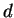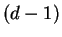Next: What is 0-1 polytope? Up: Convex Polyhedron Previous: What is cube/hypercube/cross polytope?   Contents

## What is simple/simplicial polytope?

A-polytope is called simple if each vertex is contained in exactlyfacets. A-polytope is called simplicial if each facet contains exactlyvertices. By definition, a dual of simple (simlicial) polytope is simplicial (simple, respectively). Every facet of a simplicial-polytope is a-simplex. Each vertex of a simple-polytope is contained in exactly-edges.

A-cube is a simple polytope and a-simplex is both simple and simplicial.

Komei Fukuda 2004-08-26